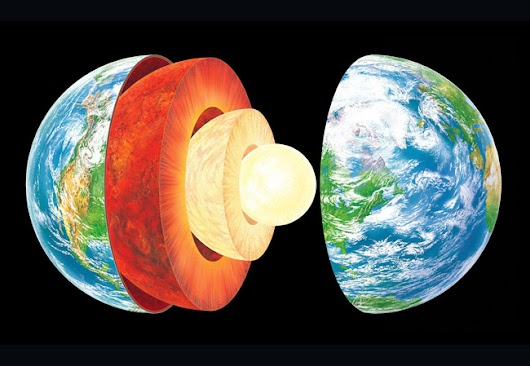Earth's structure & plate margins - Quiz 1
You will be instantly emailed feedback on submitting the quiz.
You can resubmit the quiz as many times as you wantFor example, Joe Bloggs
Which academy do you attend? *
Which year group are you currently in? *
Which class are you in? *
Which of the following statements are true?
2 points
The Earth is split into .... main layers. *
1 point
The section of the Earth right in the centre is called the... *
1 point
The section of the Earth on the surface (around 20km thick) is called the... *
1 point
Which of the following layers are molten or semi-molten (liquid)? *
2 points
Required
Which of the following statements about the Earth's internal structure are correct? *
3 points
Required
The two types of tectonic crust are... *
2 points
Required
The continental crust is... *
3 points
Required
Which of the following sequences is correct for convection currents? *
1 point
The point where two tectonic plates meet is called: *
1 point
The following are types of tectonic plate boundaries: *
3 points
Required
When the two plates move towards each other, the plate boundary is called... *
1 point
When the two plates away from each other, the plate boundary is called... *
1 point
When the two plates slide past each other (in the same direction or different directions), the plate boundary is called... *
1 point
At a destructive plate boundary, which of the following statements are correct? *
6 points
Required
At a constructive plate boundary, which of the following statements are correct? *
2 points
Required
At a conservative plate boundary, which of the following statements are correct? *
4 points
Required
Which of the following is an example of a destructive plate boundary? *
1 point
Which of the following is an example of a conservative plate boundary? *
1 point
An earthquake is... *
1 point
A volcano is... *
1 point
Earthquakes occur at... *
1 point
Volcanoes occur at... *
3 points
Required
What type of volcano is found at a destructive plate margin? *
1 point
The point in the Earth where the earthquake occurs is called the... *
1 point
The point on the Earth's surface straight above where the earthquake occurs is called the... *
1 point